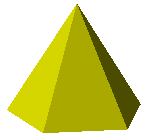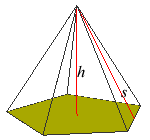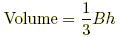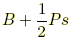index: click on a letter A B C D E F G H I J K L M N O P Q R S T U V W X Y Z A to Z index index: subject areas numbers & symbols sets, logic, proofs geometry algebra trigonometry advanced algebra & pre-calculus calculus advanced topics probability & statistics real world applications multimedia entrieswww.mathwords.com about mathwords website feedback

Right Regular Pyramid

A right pyramid with a base that is a regular polygon. If the base is a triangle then the figure is called a tetrahedron.

Note: For some mathematicians regular pyramid means the same as right regular pyramid.

Additional note: The Egyptian pyramids are right regular pyramids with square bases.Solid view: right regular pyramid with a regular pentagon as baseFrame view: right regular pyramid with a regular pentagon as baseLateral surface area for a right regular pyramid =Total surface area for a right regular pyramid =h = height of the pyramid B = area of the base P = perimeter of the base s = slant height